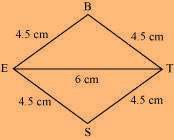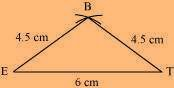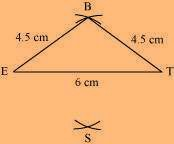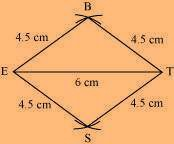# Construct the following quadrilateral: Rhombus BEST. BE = 4.5 cm ET = 6 cm

Solution

We know that all sides of a rhombus are of the same measure.

Hence, BE = ES = ST = TB The rough sketch of the rhombus BEST can be drawn as follows.(1) ∆ BET can be constructed by using the given measurements as follows.(2) Vertex S is 4.5 cm away from vertex E and also from vertex T.

Hence, while taking E and T as centres, draw arcs of 4.5 cm radius, which will be intersecting each other at point S.(3) Join S to E and T.BEST is the required rhombus.